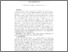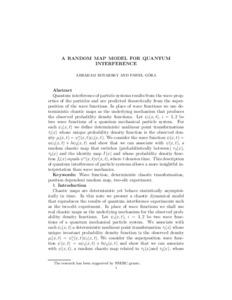Title:

# A random map model for quantum interference

Boyarsky, Abraham and GÓRA, PAWEŁ (2010) A random map model for quantum interference. Communications in Nonlinear Science and Numerical Simulation, 15 (8). pp. 1974-1979. ISSN 10075704Preview
Text (application/pdf)
gora2010b.pdf - Accepted Version
143kB

Official URL: http://dx.doi.org/10.1016/j.cnsns.2009.08.018

## Abstract

Quantum interference of particle systems results from the wave properties of the particles and are predicted theoretically from the superposition of the wave functions. In place of wave functions we use deterministic chaotic maps as the underlying mechanism that produces the observed probability density functions. Let be two wave functions of a quantum mechanical particle system. For each ψi(x,t) we define deterministic nonlinear point transformations τi(x) whose unique probability density function is the observed density . We consider the wave function ψ(x,t)=aψ1(x,t)+bψ2(x,t) and show that we can associate with ψ(x,t), a random chaotic map that switches (probabilistically between) τ1(x),τ2(x) and the identity map I(x) and whose probability density function ft(x) equals ψ∗(x,t)ψ(x,t), where t denotes time. This description of quantum interference of particle systems allows a more insightful interpretation than wave mechanics.

Divisions: Concordia University > Faculty of Arts and Science > Mathematics and Statistics Article Yes Boyarsky, Abraham and GÓRA, PAWEŁ Communications in Nonlinear Science and Numerical Simulation 2010 10.1016/j.cnsns.2009.08.018 Wave function; Deterministic chaotic transformation; Position dependent random map; Two-slit experiment 976830 DANIELLE DENNIE 29 Jan 2013 14:24 18 Jan 2018 17:43

## References:

 S. Pelikan Invariant densities for random maps of the interval Trans Amer Math Soc, 281 (1984), pp. 813–824

 P. Góra, A. Boyarsky Absolutely continuous invariant measures for random maps with position dependent probabilities J Math Anal Appl, 278 (2003), pp. 225–242

 M. Barnsley Fractals everywhere Academic Press, London (1988)

 M. McClendon, H. Rabitz Numerical simulations in stochastic mechanics Phys Rev A, 37 (9) (1998), pp. 3479–3492
All items in Spectrum are protected by copyright, with all rights reserved. The use of items is governed by Spectrum's terms of access.

Repository Staff Only: item control pageResearch related to the current document (at the CORE website)
Back to top